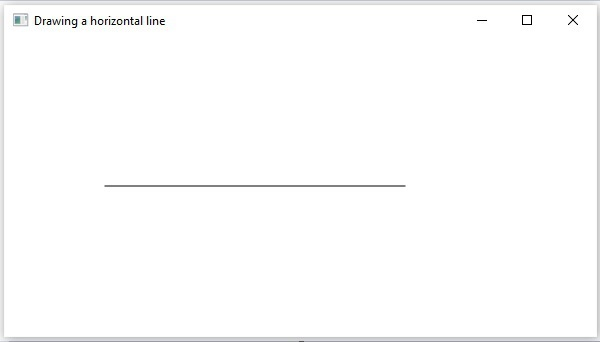# 2D Shapes PathElement Horizontal Line

The path element HLineTo is used to draw a horizontal line to a point in the specified coordinates from the current position.

It is represented by a class named HLineTo. This class belongs to the package javafx.scene.shape.

This class has a property of the double datatype namely −

• X − The x coordinate of the point to which a horizontal line is to be drawn from the current position.

To draw a path element horizontal line, you need to pass a value to this property. This can be either done by passing it to the constructor of this class, at the time of instantiation, as follows −

```HLineTO hline = new HLineTo(x);
```

Or, by using their respective setter methods as shown below −

```setX(value);
```

## Steps to draw PathElement Horizontal Line

Follow the steps given below to draw a horizontal line to a specified point from the current position in JavaFX.

### Step 1: Creating a Class

Create a Java class and inherit the Application class of the package javafx.application and implement the start() method of this class as follows.

```public class ClassName extends Application {
@Override
public void start(Stage primaryStage) throws Exception {
}
}
```

### Step 2: Instantiating the Path Class

Create the path class object as follows −

```//Creating a Path object
Path path = new Path()
```

### Step 3: Setting the Initial Point

Create the MoveTo path element and set XY coordinates to starting point of the line to the coordinates (100, 150). This can be done by using the methods setX() and setY() of the class MoveTo as shown below.

```//Moving to the starting point
MoveTo moveTo = new MoveTo();
moveTo.setX(100.0f);
moveTo.setY(150.0f);
```

### Step 4: Creating an Object of the Class HLineTo

Create the path element horizontal line by instantiating the class named HLineTo which belongs to the package javafx.scene.shape as shown below.

```//Creating an object of the class HLineTo
HLineTo hLineTo = new HLineTo();
```

### Step 5: Setting Properties to the Horizontal Line Element

Specify the x coordinate of the point to which a horizontal line is to be drawn from the current position. This can be done by setting the property x, using the method setX() of the HLineTo class as shown below.

```//Setting the Properties of the horizontal line element
hlineTo.setX(400)
```

### Step 6: Adding Elements to the Observable List of Path Class

Add the path elements MoveTo and HlineTo created in the previous steps to the observable list of the Path class as shown below −

```//Adding the path elements to Observable list of the Path class
```

### Step 7: Creating a Group Object

Create a group object by instantiating the class named Group, which belongs to the package javafx.scene.

Pass the Line (node) object created in the previous step as a parameter to the constructor of the Group class. This should be done in order to add it to the group as shown below −

```Group root = new Group(line);
```

### Step 8: Creating a Scene Object

Create a Scene by instantiating the class named Scene which belongs to the package javafx.scene. To this class, pass the Group object (root) created in the previous step.

In addition to the root object, you can also pass two double parameters representing height and width of the screen along with the object of the Group class as follows −

```Scene scene = new Scene(group ,600, 300);
```

### Step 9: Setting the Title of the Stage

You can set the title to the stage using the setTitle() method of the Stage class. The primaryStage is a Stage object which is passed to the start method of the scene class as a parameter.

Using the primaryStage object, set the title of the scene as Sample Application as follows.

```primaryStage.setTitle("Sample Application");
```

### Step 10: Adding Scene to the Stage

You can add a Scene object to the stage using the method setScene() of the class named Stage. Add the Scene object prepared in the previous steps using this method as shown below −

```primaryStage.setScene(scene);
```

### Step 11: Displaying the Contents of the Stage

Display the contents of the scene using the method named show() of the Stage class as follows.

```primaryStage.show();
```

### Step 12: Launching the Application

Launch the JavaFX application by calling the static method launch() of the Application class from the main method as follows.

```public static void main(String args[]){
launch(args);
}
```

Example

Following is a program which draws a horizontal line from the current point to a specified position using the class Path of JavaFX. Save this code in a file with the name − HLineToExample.java.

```import javafx.application.Application;
import javafx.scene.Group;
import javafx.scene.Scene;
import javafx.stage.Stage;
import javafx.scene.shape.HLineTo;
import javafx.scene.shape.MoveTo;
import javafx.scene.shape.Path;

public class HLineToExample extends Application {
@Override
public void start(Stage stage) {
//Creating an object of the Path class
Path path = new Path();

//Moving to the starting point
MoveTo moveTo = new MoveTo();
moveTo.setX(100.0);
moveTo.setY(150.0);

//Instantiating the HLineTo class
HLineTo hLineTo = new HLineTo();

//Setting the properties of the path element horizontal line
hLineTo.setX(10.0);

//Adding the path elements to Observable list of the Path class

//Creating a Group object
Group root = new Group(path);

//Creating a scene object
Scene scene = new Scene(root, 600, 300);

//Setting title to the Stage
stage.setTitle("Drawing a horizontal line");

stage.setScene(scene);

//Displaying the contents of the stage
stage.show();
}
public static void main(String args[]){
launch(args);
}
}
```

Compile and execute the saved java file from the command prompt using the following commands.

```javac HLineToExample.java
java HLineToExample
```

On executing, the above program generates a JavaFX window displaying a horizontal line, which is drawn from the current position to the specified point, as shown below.javafx_2d_shapes.htm